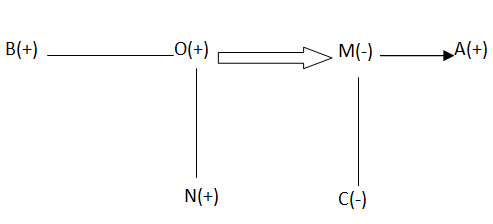# Sony Reasoning Question

DIRECTIONS for the question 1 to 5 : Read the information given below and answer the questions that follow.

A family consists of six members M, N, O and C, B and A. N is the son of O but O is not the mother of N. M and O are a married couple. B is the brother of O. C is the daughter of M.A is the brother of M

1. Who is the brother-in-law of O?
1. M
2. A
3. B
4. C
5. None of theseAs shown above A is the brother-in-law of O. Hence option B is the answer
2. Who is the father of N?
1. M
2. O
3. A
5. None of theseAs shown above O is the father of N. Hence option C is the answer.
1. How many children does M have?
1. 4
2. 3
3. 2
4. 1
5. None of theseAs shown above does M has 2 children. Hence option C is the answer.
2. How many female members are there in the family?.
1. 1
2. 2
3. 3
4. 4
5. None of theseAs shown above there are 2 female members in the family. Hence option B is the answer.
3. Which is the pair of brothers?
1. O & B
2. N & C
3. M & A
4. M & A
5. None of theseAs shown above O and B is the pair of brothers. Hence option A is the answer.
DIRECTIONS for the question 6 to 10 : Answer the following questions as per the best of your judgment.
4. In a certain code CASUAL is coded as GEWYEP. How is PEOPLE coded in that code language?
1. SHRSOH
2. TISTPI
3. SIRTOI
4. THSTPI
5. None of these

The pattern is +4 +4 and so on.
5. In a certain code language,"rit nae som" means "orange is green", "nae to tep" means "orange and white". Which of the following represents "orange" in that code language?
1. som
2. nit
3. nae
4. ro
5. None of these

Since the common code is (nae) and the common word is (orange), so nae is the code for orange
6. If "rose" is called "popy", "popy" is called "lily", "lily" is called "lotus" and "lotus" is called "jasmine", which of the following is the king of flowers?
1. ROse
2. Lotus
3. Popy
4. Lily
5. Jasmine

The king of flowers is Rose and Rose is called Popy.
7. "Knowledge" is related to "Ignorance" in the same way as "intelligent" is related to
1. Brilliant
2. Dull
3. Light
4. Fast
5. Accurate